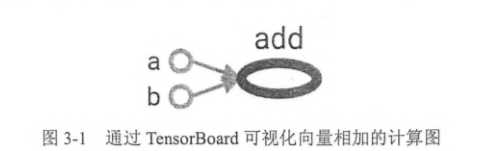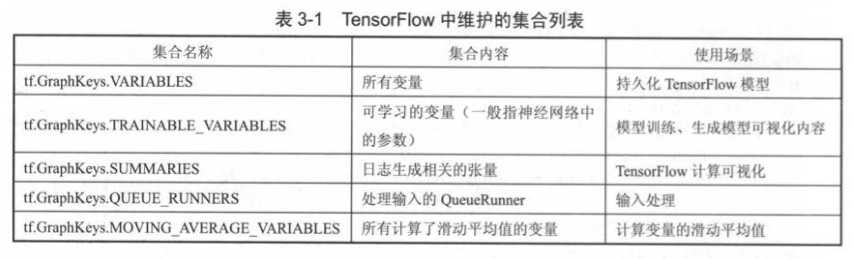# Tensorflow计算模型——计算图

1.计算图的概念：

计算图：输入和计算函数都以节点的形式出现，而节点的输出项之间的关系以有向线段表示所构成的计算图形。2. 计算图的使用：

```import tensorflow as tf

‘‘‘tensorflow程序第一步：定义计算图中所有计算‘‘‘
a=tf.constant([1.0, 2.0], name="a")
b=tf.constant([2.0, 3.0], name="b")
result=a+b

‘‘‘判断a节点是不是在默认节点中‘‘‘
print(a.graph is tf.get_default_graph())
print(b.graph is tf.get_default_graph())

True
True```

```import tensorflow as tf

‘‘‘tensorflow第一步：定义所有计算‘‘‘

g1=tf.Graph()
with g1.as_default():
‘‘‘在计算图g1中定义变量“v"，并初始化为0‘‘‘
v=tf.get_variable( "v", shape=[1,2],initializer=tf.zeros_initializer )

g2=tf.Graph()
with g2.as_default():
‘‘‘在计算图g2中定义变量“v"，初始化为1‘‘‘
v=tf.get_variable( "v", shape=[2,3],initializer=tf.ones_initializer )

‘‘‘tensorflow第二步：运行计算‘‘‘

with tf.Session(graph=g1) as sess:
tf.initialize_all_variables().run()         #初始化所有变量
with tf.variable_scope("", reuse=True):
print(sess.run(tf.get_variable("v")))       #获取变量v

with tf.Session(graph=g2) as sess:
tf.initialize_all_variables().run()
with tf.variable_scope("", reuse=True):
print(sess.run(tf.get_variable("v")))

[[0. 0.]]
[[1. 1. 1.]
[1. 1. 1.]]```

tf.get_collection函数，获取指定collection中的所有资源

几个常用的集合Tensorflow计算模型——计算图

(0)
(0)